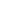Share

# Binary

Binary is a two-digit (Base-2) numerical system, which computers use to store data and compute functions. The reason computers use the binary system is because digital switches inside the computer can only be set to either on or off, which are represented by a 1 or 0. Though the binary system consists of only ones and zeros, the two digits can be used to represent any number.

For example:

A single 0 in binary represents zero.
A single 1 represents (2^0) or 1.
10 represents (2^1) or 2.
11 represents (2^1 + 2^0) or 3.
100 represents (2^2) or 4.
101 represents (2^2 + 2^0) or 5.
110 represents (2^2 + 2^1) or 6.
111 represents (2^2 + 2^1 + 2^0) or 7.
1000 represents (2^3) or 8, and so on.

Published: 2002

Definition from the PC Glossary
https://pc.net/glossary/binary